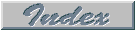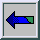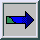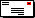## Flow Through Packed Beds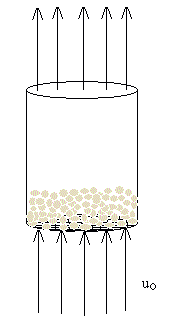This image depicts the flow of a fluid through a pipe containing small spherical particles. The fluid flows at some superficial velocity (uo), as measured upstream of the pipe entrance. This situation is termed "flow through a packed bed".

Fluid flowing with velocity uo through a Packed Bed

Flow through a packed bed can be regarded as fluid flow past some number of submerged objects. In this case, the objects are uniform spherical particles of diameter dp.

When there is no flow through the packed bed, the net gravitational force (including bouyancy) acts downward. When flow begins upward, friction forces act upward and counterbalance the net gravitational force. For a high enough fluid velocity, the friction force is large enough to lift the particles. This represents the onset of fluidization. The frictional force can be expressed in terms of a friction factor. This leads to equations describing the the flow of a fluid past a collection of particles. The Ergun equation is one such equation.

#### Flow through a packed bed can be described by the Ergun Equation.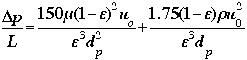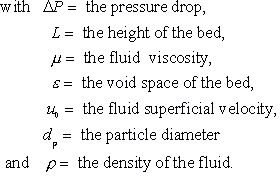The Ergun equation tells us a number of things. It tells us the pressure drop along the length of the packed bed given some fluid velocity. It also tells us that the pressure drop depends on the packing size, length of bed, fluid viscosity and fluid density.

#### Now on to Fluidization

• The Fluidized Bed Phenomena

• Doug Thornhill